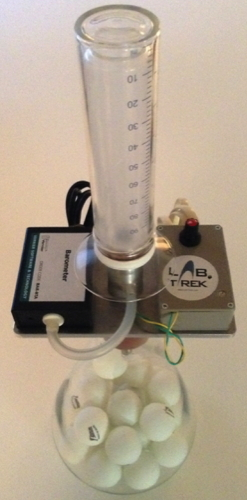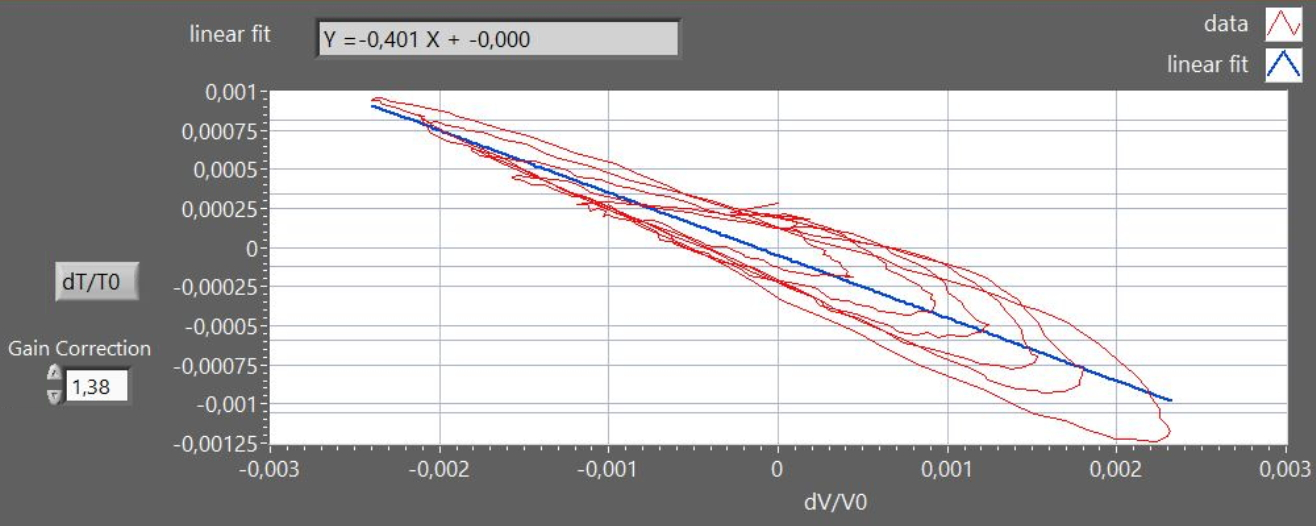Rüchardt's experiment for measuring γ=cp/cv in airThe apparatus (figure 1) allows to measure the ratio γ=Cp/Cv for air, using a modern version of the Rüchardt method, where Cp=specific heat at constant pressure and Cv=specific heat at constant volume. With this apparatus one simultaneously measures the changes of three thermodynamic variables (pressure, volume and temperature) during the fast volume oscillations induced in a mass of air by a low-friction sliding piston (adiabatic transformation). Volume is measured by a motion sensor (sonar), pressure is measured by a pressure sensor (barometer),  temperature is measured by a special sensor that monitors the resistance of a  tungsten wire . In adiabatic regime, where the Poisson relation holds (PVγ=cost), the γ value may be obtained not only, as in classic Rüchardt method, from the measured oscillation period, but also from various  P-V-T plots. For small changes in pressure  dP and volume dV the Poisson relation is dP= -(γP/V)dV A small displacement x of the piston from the equilibrium position, produces a volume change dV = xA (where A is the piston cross section) and a restoring force :  F =A dP = - A2 x (γ P/V). The free piston oscillations describes therefore a (damped) harmonic motion (figure 2). A plot of the reduced variables dP/P0 vs. dV/V0  (figure 3) is a straight line with slope - γ. By substituting P=nRT/V into the Poisson equation we get the relation TV(γ-1) = const, from which : dT/T= (γ-1) dV/V which allows to evaluate γ from  a plot of dT/T vs. dV/V : in figure 4, the graph shows a Lissajous curve approximating an elliptical spiral due to the time-lag in the thermometer response. Moreover, in figure 4 the nominal thermometer output was corrected by a factor 1.38, to obtain the expected slope value (-0.4), accounting for the attenuation of the signal due to the intrinsic low-pass filter provided by the thermometer finite heat-capacity. The dynamic may be varied by changing the value of the oscillating mass m (by loading the piston with metal rings) or the effective air volume V (by inserting ping-pong balls into the vessel ). see: A new MBLversion of the Rüchardt experiment Am. J. Phys. 69, (2001)Figure 1Figure 2Figure 3Figure 4